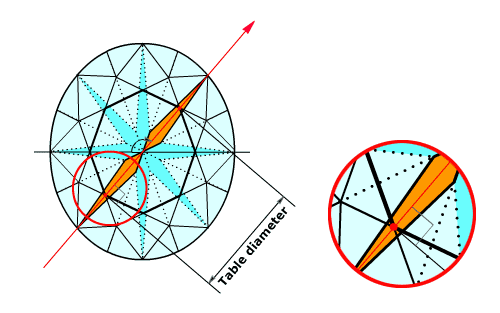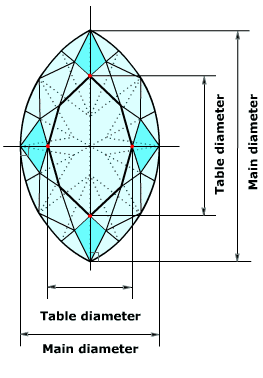Helium help : Comprehensive list of report parametersTable diametersApplicable to: Brilliant Rounded Fancies / Marquise, Oval, Pear Rectangular Fancies / Princess, Radiant StepCuts / Emerald, Triangle Unknown cuts The diameter of the table facet in some given direction is measured as a distance between two parallel vertical planes placed perpendicularly to the given direction as close to each other as possible, so that all vertices of the table facet lay between these planes (similarly to usual diameter). Brilliant There are 4 diameters of the table. These diameters are measured using main pavilion facets for determining the directions of measurement. Azimuths of each pair of opposite main pavilion facets define the direction, in which the table diameter is measured. All relative table diameters are calculated using average model diameter. The software calculates average, minimum, maximum table values using these 4 parameters.Rounded Fancies (Marquise, Oval, Pear) Only two diameters of the table are measured for the rounded fancies cuts: along minimum and maximum diameters. The table is measured using main crown facets for determining the directions of measurement. Azimuths of two pairs of opposite main crown facets define the direction, in which the table diameter is measured. The relative table diameters are calculated using main diameters measured in the same direction. The software calculates average, minimum, maximum table values using these 2 parameters.